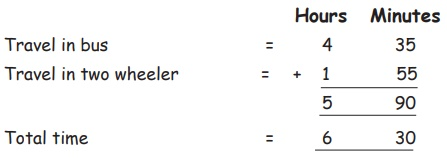Home | | Maths 5th Std | Use addition and subtraction in finding time interval

# Use addition and subtraction in finding time interval

Use addition and subtraction in finding time interval

Use addition and subtraction in finding time interval

Example

Add: 4 hours 30 minutes and 2 hours 45 minutes

75 minutes = 1 hour 15 minutes7 Hour 15 minutes

Example

Krishna goes to his village. He travels 4 hours 35 minutes in bus and 1 hour 55 minutes in two wheeler. What is his total time of travel?Kirshna travels totally for 6 hour 30 minutes

90 minutes = 60+30 minutes

60 minutes = 1 hour

30 minutes = 30 minutes

5+1 = 6 hour 30 minutes

Subtraction

Example

Subtract : 3 hours 45 minutes from 5 hours 30 minutesWe can’t Subtract 45 minutes from 5 hours 30 minutes so we change the 5 hours in to (60 + 30) 90 minutes and subtract 45 minutes from 90 minutes. We can get 45 minutes.

When we subtract 3 hours from 4 hours balance is 1 hour

Example

Ram works on his Computer from morning 10' O Clock to Evening 3.30. How long does he work on his computer?

Ram work on his Computer at Evening = 3 Hours 30 Minutes

His work at Morning = 10 Hours 00 MinutesSubtract from a.m. to p.m., we add 12 hours.

Add 3.30 hours + 12 hours we can get 15:30

Ram works 5 hours 30 minutes on his computer

Example1. Find the time interval between First bell and morning break.The time interval between First bell and morning break is 2 Hours.

2. Find the time interval between the time of class starting and the time of morning break.The time interval between class starting time and morning break ending time is 1 hour 40 minutes.

We can’t subtract 30 minutes from 10 minutes so we convert to hour into minutes 1 hours to 60 minutes and add.

60+10 = 70 now we subtract 30 minutes from 40 we can get 1 hour 40 minutes.

3. Find the total time from starting of morning class to Closing of Afternoon class.Add 12 hours, 4.10 + 12.00 = 16 Hours + 10 minutes

The total time from starting of morning class to closing of afternoon class is 6 hours 40 minutes.

Try this

Find the time interval between starting of afternoon class and closing of evening class.Subtract from am to pm, we add 12 hours and then subtract the a.m time.

Tags : Time | Term 1 Chapter 5 | 5th Maths , 5th Maths : Term 1 Unit 5 : Time
Study Material, Lecturing Notes, Assignment, Reference, Wiki description explanation, brief detail
5th Maths : Term 1 Unit 5 : Time : Use addition and subtraction in finding time interval | Time | Term 1 Chapter 5 | 5th Maths